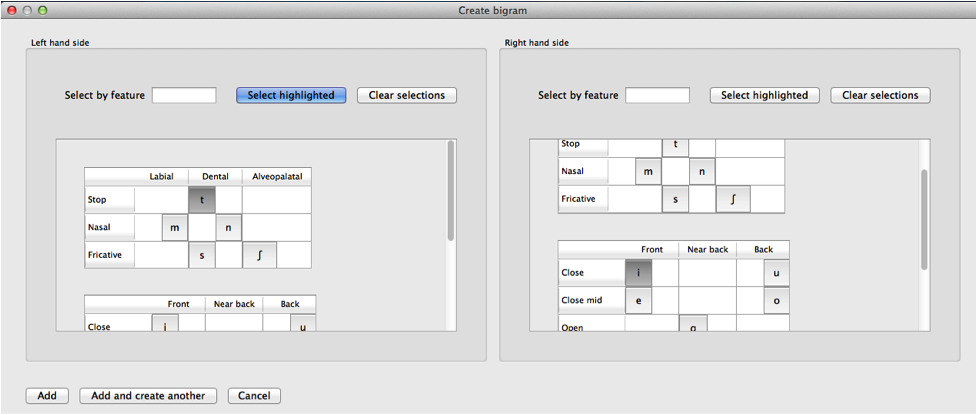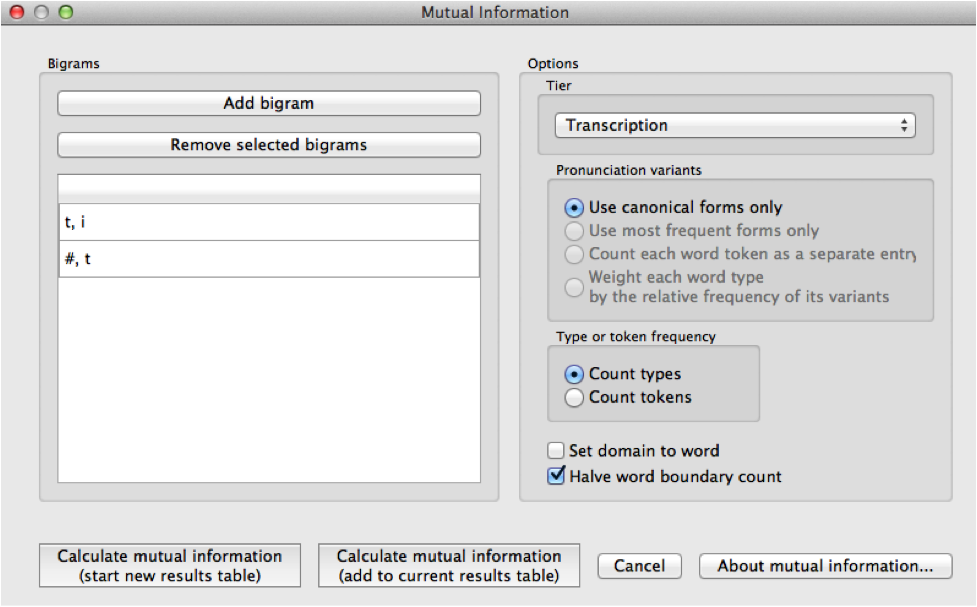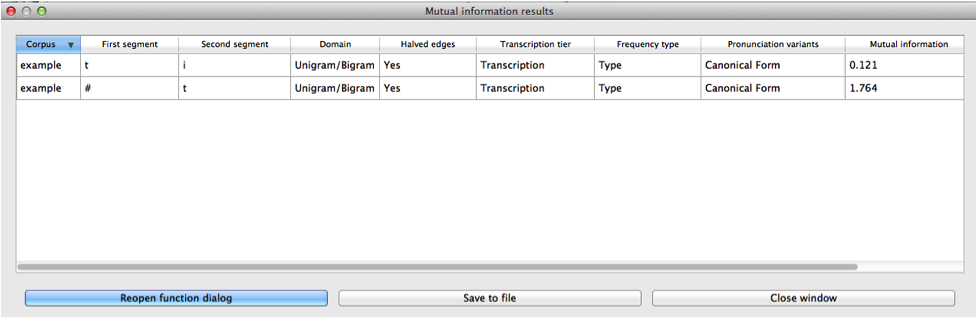# Mutual Information¶

Mutual information  is a measure of how much dependency there is between two random variables, X and Y. That is, there is a certain amount of information gained by learning that X is present and also a certain amount of information gained by learning that Y is present. But knowing that X is present might also tell you something about the likelihood of Y being present, and vice versa. If X and Y always co-occur, then knowing that one is present already tells you that the other must also be present. On the other hand, if X and Y are entirely independent, then knowing that one is present tells you nothing about the likelihood that the other is present.

In phonology, there are two primary ways in which one could interpret X and Y as random variables. In one version, X and Y are equivalent random variables, each varying over “possible speech sounds in some unit” (where the unit could be any level of representation, e.g. a word or even a non-meaningful unit such as a bigram). In this case, one is measuring how much the presence of X anywhere in the defined unit affects the presence of Y in that same unit, regardless of the order in which X and Y occur, such that the mutual information of (X; Y) is the same as the mutual information of (Y; X), and furthermore, the pointwise mutual information of any individual value of each variable (X = a; Y = b) is the same as the pointwise mutual information of (X = b; Y = a). Although his is perhaps the most intuitive version of mutual information, given that it does give a symmetric measure for “how much information does the presence of a provide about the presence of b,” we are not currently aware of any work that has attempted to use this interpretation of MI for phonological purposes.

The other interpretation of MI assumes that X and Y are different random variables, with X being “possible speech sounds occurring as the first member of a bigram” and Y being “possible speech sounds occurring as the second member of a bigram.” This gives a directional interpretation to mutual information, such that, while the mutual information of (X; Y) is the same as the mutual information of (Y; X), the pointwise mutual information of (X = a; Y = b) is NOT the same as the pointwise mutual information of (X = b; Y = a), because the possible values for X and Y are different. (It is still, trivially, the case that the pointwise mutual information of (X = a; Y = b) and (Y = b; X = a) are equal.)

This latter version of mutual information has primarily been used as a measure of co-occurrence restrictions (harmony, phonotactics, etc.). For example, [Goldsmith2012] use pointwise mutual information as a way of examining Finnish vowel harmony; see also discussion in [Goldsmith2002]. Mutual information has also been used instead of transitional probability as a way of finding boundaries between words in running speech, with the idea that bigrams that cross word boundaries will have, on average, lower values of mutual information than bigrams that are within words (see [Brent1999], [Rytting2004]). Note, however, that in order for this latter use of mutual information to be useful, one must be using a corpus based on running text rather than a corpus that is simply a list of individual words and their token frequencies.

## Method of calculation¶

Both of the interpretations of mutual information described above are implemented in PCT. We refer to the first one, in which X and Y are interpreted as equal random variables, varying over “possible speech sounds in a unit,” as word-internal co-occurrence pointwise mutual information (pMI), because we specifically use the word as the unit in which to measure pMI. We refer to the second one, in which X and Y are different random variables, over either the first or second members of bigrams, as ordered pair pMI.

The general formula for pointwise mutual information is given below; it is the binary logarithm of the joint probability of X = a and Y = b, divided by the product of the individual probabilities that X = a and Y = b.

$$pMI = log_2 (\frac{p(X=a \& Y = b)}{p(X=a)*p(Y=b)})$$

Word-internal co-occurrence pMI: In this version, the joint probability that X = a and Y = b is equal to the probability that some unit (here, a word) contains both a and b (in any order). Therefore, the pointwise mutual information of the sounds a and b is equal to the binary logarithm of the probability of some word containing both a and b, divided by the product of the individual probabilities of a word containing a and a word containing b.

Pointwise mutual information for individual segments:

$$pMI_{word-internal} = log_2 (\frac{p(a \in W \& b \in W)} {p(a \in W)*p(b \in W)})$$

Ordered pair pMI: In this version, the joint probability that X = a and Y = b is equal to the probability of occurrence of the sequence ab. Therefore, the pointwise mutual information of a bigram (e.g., ab) is equal to the binary logarithm of the probability of the bigram divided by the product of the individual segment probabilities, as shown in the formula below.

Pointwise mutual information for bigrams:

$$pMI_{ordered-pair} = log_2 (\frac{p(ab)} {p(a)*p(b)})$$

For example, given the bigram [a, b], its pointwise mutual information is the binary logarithm of the probability of the sequence [ab] in the corpus divided by a quantity equal to the probability of [a] times the probability of [b]. Bigram probabilities are calculated by dividing counts by the total number of bigrams, and unigram probabilities are calculated equivalently.

Note that pMI can also be expressed in terms of the information content of each of the members of the bigram. Information is measured as the negative log of the probability of a unit $$(I(a) = -log_2*p(a))$$, so the pMI of a bigram ab is also equal to $$I(a) + I(b) – I(ab)$$.

Note that in PCT, calculations are not rounded until the final stage, whereas in [Goldsmith2012], rounding was done at some intermediate stages as well, which may result in slightly different final pMI values being calculated.

## Calculating mutual information in the GUI¶

To start the analysis, click on “Analysis” / “Calculate mutual information...” in the main menu, and then follow these steps:

1. Bigram: Click on the “Add bigram” button in the “Mutual Information” dialogue box. A new window will open with an inventory of all the segments that occur in your corpus. Select the bigram by clicking on one segment from the “left-hand side” and one segment from the “right-hand side.” Note that the order of the sounds matters in this function! To add more than one bigram, click “Add and create another” to be automatically returned to the selection window. Once the last bigram has been selected, simply click “Add” to return to the Mutual Information dialogue box. All the selected bigrams will appear in a list. To remove one, click on it and select “Remove selected bigram.”
2. Tier: Mutual information can be calculated on any available tier. The default is transcription. If a vowel tier has been created, for example, one could calculate the mutual information between vowels on that tier, ignoring intervening consonants, to examine harmony effects.
3. Pronunciation variants: If the corpus contains multiple pronunciation variants for lexical items, select what strategy should be used. For details, see Pronunciation Variants.
4. Type vs. Token Frequency: Next, pick whether you want the calculation to be done on types or tokens, assuming that token frequencies are available in your corpus. If they are not, this option will not be available. (Note: if you think your corpus does include token frequencies, but this option seems to be unavailable, see Required format of corpus on the required format for a corpus.)
5. Domain: Choosing “set domain to word” will change the calculation so that the calculation is for word-internal co-occurrence pMI. In this case, the order and adjacency of the bigram does not matter; it is simply treated as a pair of segments that could occur anywhere in a word.
6. Word boundary count: A standard word object in PCT contains word boundaries on both sides of it (e.g., [#kæt#] ‘cat’). If words were concatenated in real running speech, however, one would expect to see only one word boundary between each pair of words (e.g., [#mai#kæt#] ‘my cat’ instead of [#mai##kæt#]). To reproduce this effect and assume that word boundaries occur only once between words (as is assumed in [Goldsmith2012], choose “halve word boundary count.” Note that this technically divides the number of boundaries in half and then adds one, to compensate for the extra “final” boundary at the end of an utterance. (It will make a difference only for calculations that include a boundary as one member of the pair.)
7. Results: Once all options have been selected, click “Calculate mutual information.” If this is not the first calculation, and you want to add the results to a pre-existing results table, select the choice that says “add to current results table.” Otherwise, select “start new results table.” A dialogue box will open, showing a table of the results, including sound 1, sound 2, the tier used, and the mutual information value. To save these results to a .txt file, click on “Save to file” at the bottom of the table.

The following image shows the inventory window used for selecting bigrams in the sample corpus:The selected bigrams appear in the list in the “Mutual Information” dialogue box:The resulting mutual information results table:To return to the function dialogue box with your most recently used selections, click on “Reopen function dialog.” Otherwise, the results table can be closed and you will be returned to your corpus view.

## Implementing the mutual information function on the command line¶

In order to perform this analysis on the command line, you must enter a command in the following format into your Terminal:

pct_mutualinfo CORPUSFILE [additional arguments]


...where CORPUSFILE is the name of your *.corpus file. If not calculating the mutal informations of all bigrams (using -l), the query bigram must be specified using -q, as -q QUERY. The bigram QUERY must be in the format s1,s2 where s1 and s2 are the first and second segments in the bigram. You may also use command line options to change the sequency type to use for your calculations, or to specify an output file name. Descriptions of these arguments can be viewed by running pct_mutualinfo -h or pct_mutualinfo --help. The help text from this command is copied below, augmented with specifications of default values:

Positional arguments:

corpus_file_name

Name of corpus file

Mandatory argument group (call must have one of these two):

-q QUERY
--query QUERY

Bigram or segment pair, as str separated by comma

-l
--all_pairwise_mis

Flag: calculate MI for all orders of all pairs of segments

Optional arguments:

-h
--help

Show help message and exit

-c CONTEXT_TYPE
--context_type CONTEXT_TYPE

How to deal with variable pronunciations. Options are ‘Canonical’, ‘MostFrequent’, ‘SeparatedTokens’, or ‘Weighted’. See documentation for details.

-s SEQUENCE_TYPE
--sequence_type SEQUENCE_TYPE

The attribute of Words to calculate MI over. Normally, this will be the transcription, but it can also be the spelling or a user-specified tier.

-o OUTFILE
--outfile OUTFILE

Name of output file

EXAMPLE 1: If your corpus file is example.corpus (no pronunciation variants) and you want to calculate the mutual information of the bigram ‘si’ using defaults for all optional arguments, you would run the following command in your terminal window:

pct_mutualinfo example.corpus -q s,i


EXAMPLE 2: Suppose you want to calculate the mutual information of the bigram ‘si’ on the spelling tier. In addition, you want the script to produce an output file called output.txt. You would need to run the following command:

pct_mutualinfo example.corpus -q s,i -s spelling -o output.txt


EXAMPLE 3: Suppose you want to calculate the mutual information of all bigram types in the corpus. In addition, you want the script to produce an output file called output.txt. You would need to run the following command:

pct_mutualinfo example.corpus -l -o output.txt


## Classes and functions¶

For further details about the relevant classes and functions in PCT’s source code, please refer to Mutual information.

  The algorithm in PCT calculates what is sometimes referred to as the “pointwise” mutual information of a pair of units X and Y, in contrast to “mutual information,” which would be the expected average value of the pointwise mutual information of all possible values of X and Y. We simplify to use “mutual information” throughout.